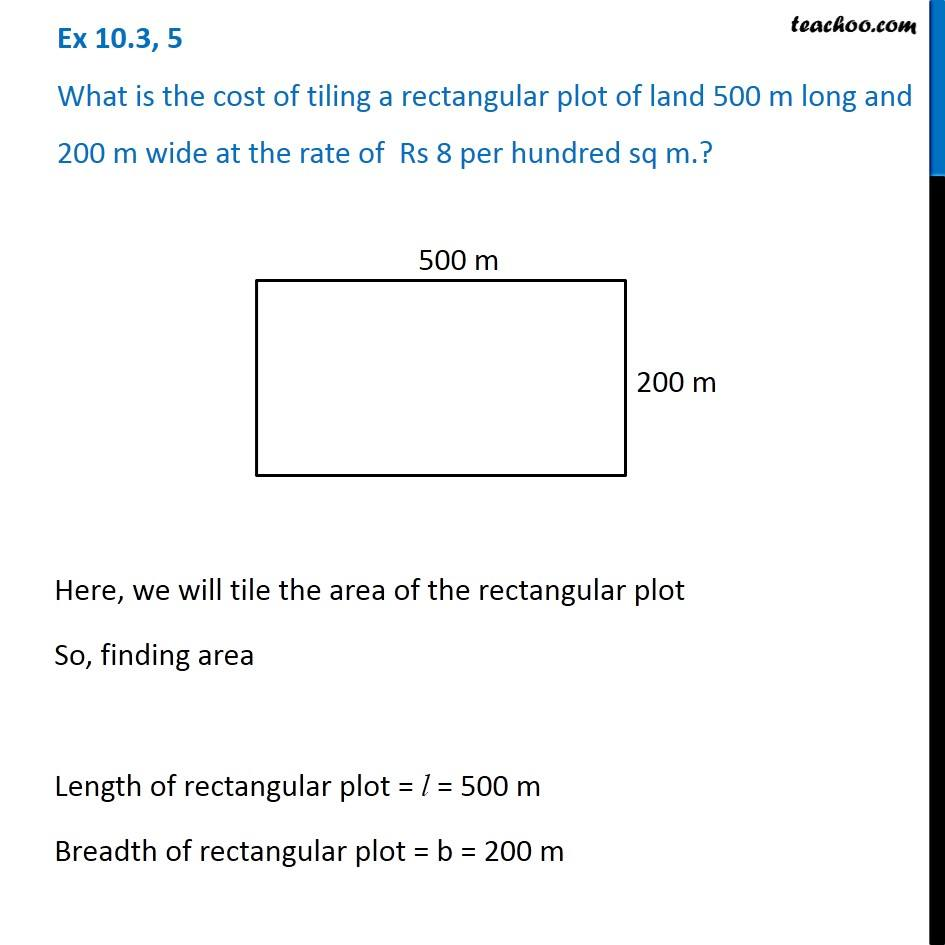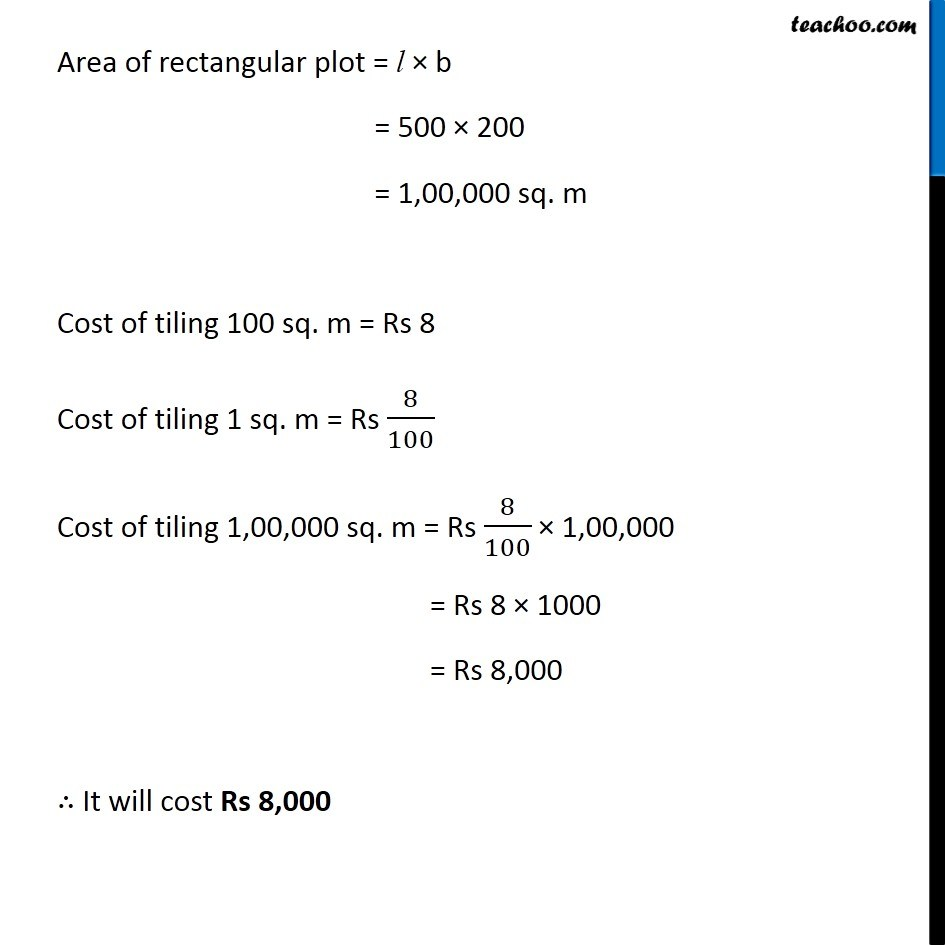Ex 10.3

Chapter 10 Class 6 Mensuration
Serial order wiseLearn in your speed, with individual attention - Teachoo Maths 1-on-1 Class

### Transcript

Ex 10.3, 5 What is the cost of tiling a rectangular plot of land 500 m long and 200 m wide at the rate of Rs 8 per hundred sq m.? Here, we will tile the area of the rectangular plot So, finding area Length of rectangular plot = l = 500 m Breadth of rectangular plot = b = 200 m Area of rectangular plot = l × b = 500 × 200 = 1,00,000 sq. m Cost of tiling 100 sq. m = Rs 8 Cost of tiling 1 sq. m = Rs 8/100 Cost of tiling 1,00,000 sq. m = Rs 8/100 × 1,00,000 = Rs 8 × 1000 = Rs 8,000 ∴ It will cost Rs 8,000